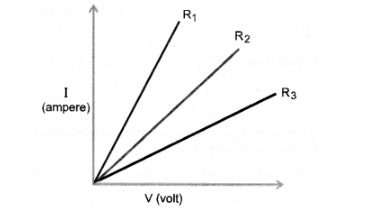# A student carries out an experiment and

Question:

A student carries out an experiment and plots the V—I graph of three samples of nichrome wire with resistances R1, R2 and R3 respectively as shown

in figure. Which of the following is true ?

(a) R1 = R2 = R3

(b) R1 > R2 > R3

(c) R3 > R2 > R1

(d) R2 > R3 > R1Solution:

(c).

Explanation: Resistance of wire $\propto \frac{1}{\text { Slope of V-Igraph }}$ Since

slope of V-I graph for $R_{3}$ is smallest, so $R_{3}$ is the highest.